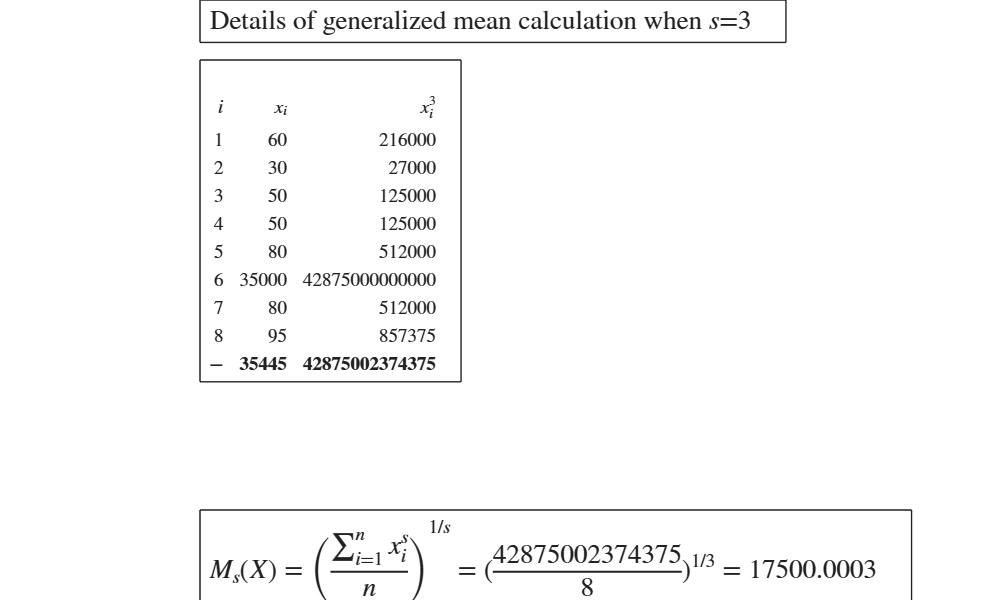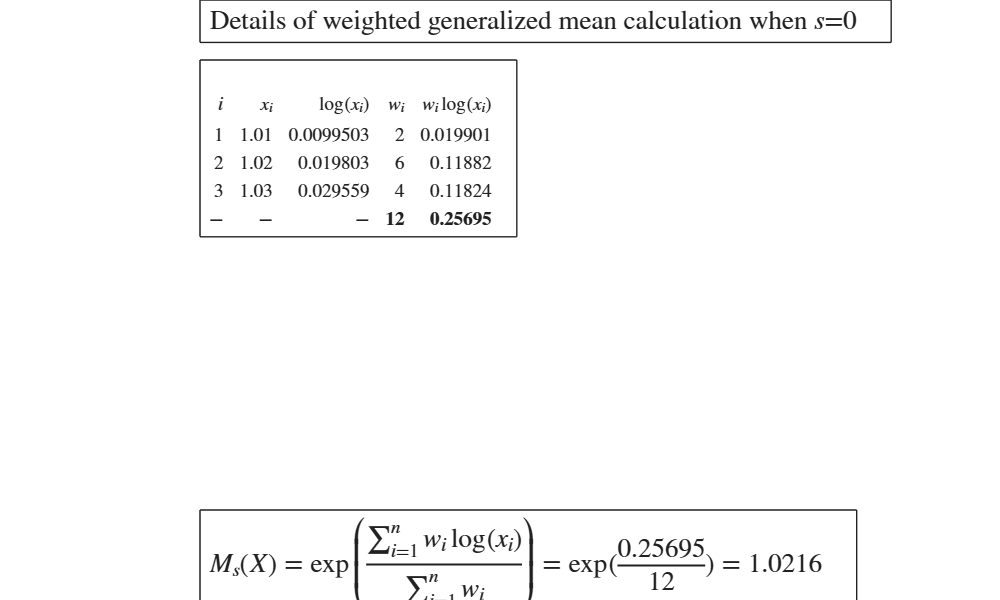GUIpowermean

GUIpowermean shows the necessary calculations to obtain the power (generalized) mean in a GUI.

Description

The power mean (also known as generalized mean, or Holder mean or Kolmogorov-Negumo function of the mean), is an abstraction of the Pythagorean means. It includes the harmonic $(s=-1)$, geometric ($s\rightarrow 0$), and arithmetic ($s=1$), quadratic ($s=2$), cubic mean ($s=3$). When $s \rightarrow -\infty$ the power mean tends to $x_{min}$ and when $s\rightarrow +\infty$ the power mean tends to $x_{max}$. http://en.wikipedia.org/wiki/Generalized_mean

out =GUIpowermean(x, s) Example of use of the cubic mean.

out =GUIpowermean(x, s, w) Example of use of weighted geometric mean.

Examples

expand allExample of use of the cubic mean.

See page 92 of [MRZ]

x=[60 30 50 50  80 35000  80 95];
out=GUIpowermean(x,3);Example of use of weighted geometric mean.

See page 82 of [MRZ]

x=[1.01 1.02 1.03];
w=[2 6 4];
out=GUIpowermean(x,0,w);Input Arguments

x — vector of numeric data. Vector.

Vector containing strictly numerical data.

Data Types: double

s — power indicator. Scalar.

Power indicator for the desired mean ($s=- \inf= x_{min}$; $s=-1$ harmonic mean; $s=0$ geometric mean; $s=1$ = arithmetic mean; $s=2$ quadratic mean; $s=3$ cubic mean, $s=+\inf$ = $x_{max}$).

Example - 1:10

Data Types: double

w — weights. Vector.

Vector of the same length of x containing the weights assigned to each observation. If w is not supplied we assume that all observations have weight equal to 1.

Example: 1:10

Data Types: double

Output Arguments

out —detailed output to compute the index. Table

Table with n+1 rows (where n is the length of x) containing what is shown in the GUI. Last row contains the totals.

Milioli, M.A., Riani, M., Zani, S. (2019), "Introduzione all'analisi dei dati statistici (Quarta edizione ampliata)". [MRZ]